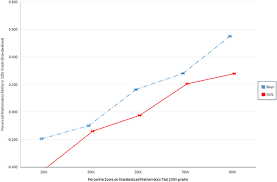## How to Calculate and Solve for TEX | Denier | Polymer & TextileThe image above represents TEX | Denier.

To compute for TEX | Denier, one essential parameter is needed and this parameter is denier (D).

The formula for calculating the TEX | Denier:

TEX = Denier / 9

Where;

TEX = TEX
D = Denier

Let’s solve an example;
Given that denier is 12. Find the TEX | Denier?

This implies that;

D = Denier = 12

TEX = Denier / 9
TEX = 12 / 9
TEX = 1.33

Therefore, the TEX is 1.33.